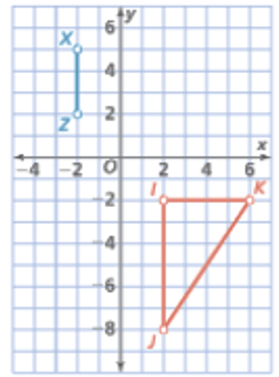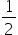Mathematics
Easy

Question

# Choose a point Y that will complete Triangle XZY so that it is similar to Triangle IJK. List the scale factor from Triangle IJK to Triangle XZY.## (-4, 5) with a scale factor of 2(-4, 5) with a scale factor of(-4, 2) with a scale factor of 2(-4, 2) with a scale factor ofHint:

## The correct answer is: (-4, 5) with a scale factor of### Related Questions to study#### With Turito Foundation.#### Get an Expert Advice From Turito.